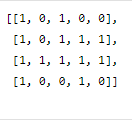Problem title
Difficulty
Avg time to solve

Moderate
30 mins
Leftmost & Rightmost Nodes of Binary Tree
Easy
20 mins
Nearest Multiple of 10
Easy
15 mins
Reverse Alternate Nodes of a Singly Linked List
Moderate
--
Single Number III
Moderate
40 mins
Lexicographical order
Moderate
35 mins
Add First and Second Reversed Half
Moderate
42 mins
Minimum Operations
Easy
20 mins
Missing Numbers
Easy
28 mins
State Diagram
Moderate
40 mins4

# Maximum Area Square

Difficulty: MEDIUM
Avg. time to solve
10 min
Success Rate
90%

Problem Statement

#### For example, for the following grid:``````The area of the largest square submatrix with all 1s is 4.
``````
##### Input Format:
``````The first line contains an integer ‘T’ denoting the number of test cases. Then each test case follows.

The first input line of each test case contains two space-integers ‘N’ and ‘M’ representing the number of rows and columns of the grid, respectively.

From the second line of each test case, the next N lines represent the rows of the grid. Every row contains M single space-separated integers.
``````
##### Output Format:
``````For each test case, print the area of maximum size square sub-matrix with all 1s.

Print the output of each test case in a separate line.
``````
##### Note:
``````You are not required to print the expected output; it has already been taken care of. Just implement the function.
``````
##### Constraints:
``````1 <= T <= 100
1 <= N <= 50
1 <= M <= 50
0 <= MAT[i][j] <= 1

Time limit: 1 sec
``````
##### Sample Input 1:
``````1
2 2
1 0
0 0
``````
##### Sample Output 1:
``````1
``````
##### Explanation of the Sample Input 1:
``````For the given grid, the largest square with all 1s has a side of length 1. So, the area will be 1 * 1 = 1.
``````
##### Sample Input 2:
``````2
3 4
1 1 1 1
0 1 1 0
0 0 0 0
2 3
0 0 0
0 0 1
``````
##### Sample Output 2:
``````4
1
``````Console